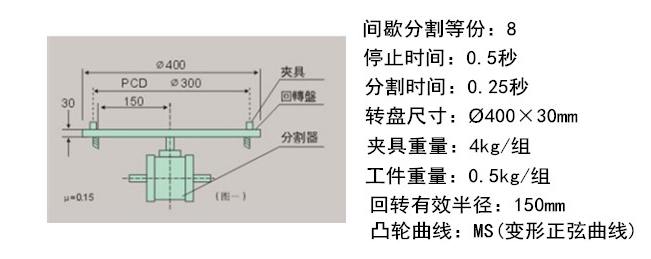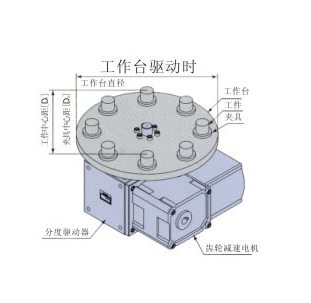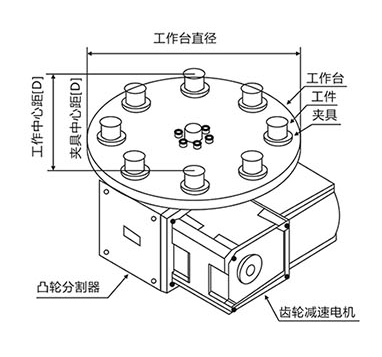# 欢迎来到山东威尔凯姆凸轮分割器厂

• 热线电话:15762561788

# 分割器选型(1)间歇分割定位等分数:S=8 S:分割数

(2)每等分驱动时间:1/3秒 定位时间:2/3秒

(3)输入轴之回转数:N=60rpm:凸轮轴速度(第分钟转速)

(4)凸轮曲线:变形修正正弦曲线

(5)回转盘的尺寸:600×16T

(6)夹具的重量:2.5kg/组

(7)工件的重量:0.3kg/组

(8)转盘依靠其底部的滑动面支持本身重量负荷,有效半径:R=0.250m

(10)驱动角:θ=360×（驱动时间）/（驱动时间+定位时间）= 120

w2=2.50×8×=20(kg) w3=0.30×8×=2.4(kg) (b) 回转盘惯性矩:I1夹具惯性矩;I2工件惯性矩;I3为 I1=w1R2/2G=35.30×0.062/2×9.8=0.16(kg..m.s) I2= w2Re2/2G=20×0.250X0.25/9.8=0.13(kg..m.s) I3=w3RRe2/2G=2.4×0.250X0.25/9.8=0.015 (kg..m.s)

(c)总惯性矩:I i=I1+I2+I3 I=0.16+0.13+0.015=0.305(kg..m.s2)

(d)输出轴最大角加速度:α=Am×2π/s×(360/θh×N/60)2=5.53×2X3.14/6×(360/120×60/60)

(1)惯性扭矩:Ti

Ti=I×α=0.305×39.09=11.92(kg . m)

(2)磨擦扭矩:Tf

Tf=μ×w×R=0.15×(35.29+20+2.4) ×0.25=2.16(Kg . m)

(3)作功扭矩:Tw

(4)以上总负载扭矩:Te

Te=Tt×Tf+Tw=11.92+2.16+0=14.08(kg . m)

Te=Tt×fe=14.08×1.5=21.12(kg . m)

Tc=360/θh .s ×Qm×Te=360/120×8 ×0.99×21.12=7.84(kg. m)

P=7.84×60 / 716×0.6=1.09(HP)或P=7.84×60 / 975×0.6 =0.804(Kw)

Vm: 最大非向性速度

Am: 最大非向性之加速度

Qm: 凸轮轴最大扭力系数

α: 角加速度

θh : 输入轴转位(驱动)

μ: 磨擦系数=0.15

γ: 速度比

Ω: 角速度(弧度/秒)

α: 角加速度(弧度/秒2)

 停止数（工位数） S 设计输入转速 ipm 每个工位的停止时间 t1 每个工位的分度时间 t2工作台直径 D1  mm 工作台重量 W1  kg 夹具P.C.D(对角夹具的中心距) D2  mm 每个夹具的重量 W2  kg 夹具的数量 n2  psc 工件P.C.D(对角夹具的中心距) D3  mm 每个工件的重量 W3  kg 工件的数量 n3  psc

### 凸轮分割器输送带结构选型数据表

 输送的单位距离 mm工件及夹具(附件)重量 kg 装有附件的链条重量 kg 链轮齿距 mm 链轮齿数 个 齿轮节圆直径 mm 齿轮外圆直径 mm 链轮重量 kg 链轮个数 个
 齿轮减速电机 需要  不需要 使用电压  V
 扭矩限制器 需要   不需要
 定时凸轮·传感器 需要   不需要